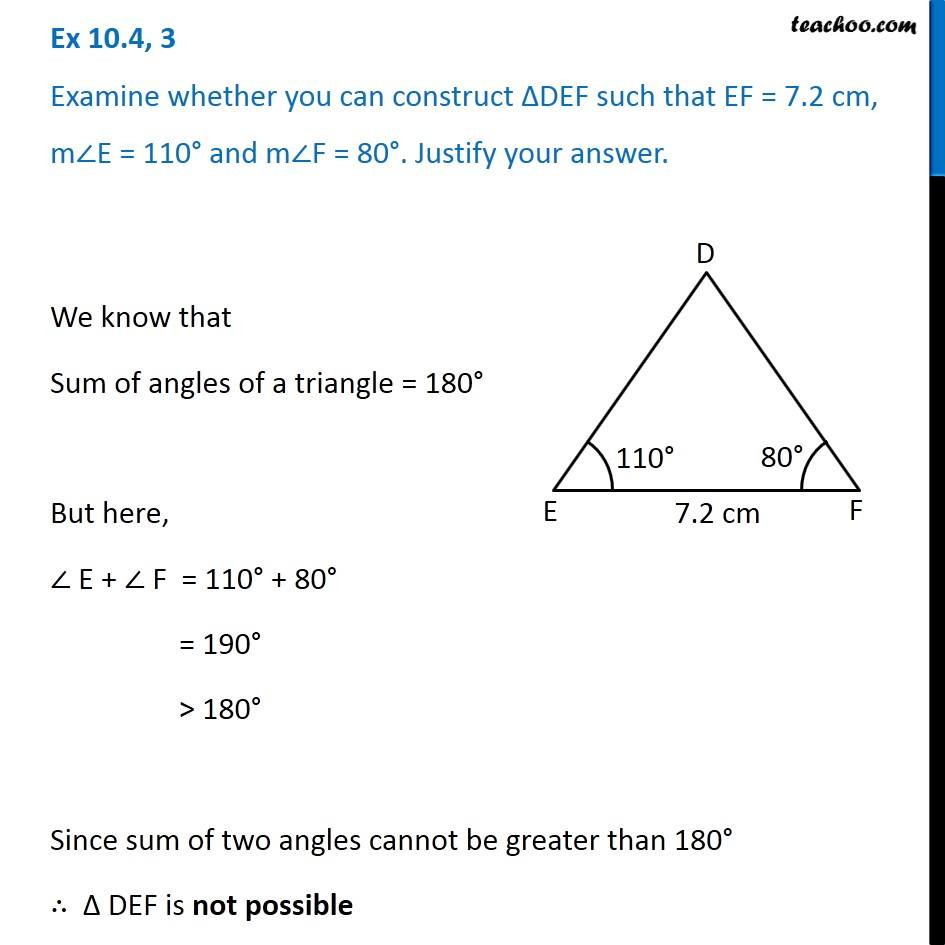Constructing a triangle using ASA congruency criteria

Practical Geometry
Serial order wiseLearn in your speed, with individual attention - Teachoo Maths 1-on-1 Class

### Transcript

Question 3 Examine whether you can construct ∆DEF such that EF = 7.2 cm, m∠E = 110° and m∠F = 80°. Justify your answer. We know that Sum of angles of a triangle = 180° But here, ∠ E + ∠ F = 110° + 80° = 190° > 180° Since sum of two angles cannot be greater than 180° ∴ Δ DEF is not possible